﻿ Tan-Cot Method to Solve (2+1)-Dimensional Bogoyavlenskii System and Konopelchenko-Dubrovsky Coupled System

### Tan-Cot Method to Solve (2+1)-Dimensional Bogoyavlenskii System and Konopelchenko-Dubrovsky Coupled ...OPEN ACCESSPEER-REVIEWED

## Tan-Cot Method to Solve (2+1)-Dimensional Bogoyavlenskii System and Konopelchenko-Dubrovsky Coupled System

Anwar Ja'afar Mohamad JawadComputer Engineering Technique Department, Al -Rafidain University College, Baghdad, Iraq

### Abstract

In this paper, we establish a traveling wave solution by using the Tan-Cot function algorithm for solving nonlinear partial differential equations. The method is used to obtain new solitary wave solutions for various type of nonlinear partial differential equations such as (2+1)-Dimensional Bogoyavlenskii system, Konopelchenko-Dubrovsky coupled system and the coupled nonlinear evolution equations which are important Soliton equations. Tan-Cot function method has been successfully implemented to establish new solitary wave solutions for the nonlinear PDEs.

• Jawad, Anwar Ja'afar Mohamad. "Tan-Cot Method to Solve (2+1)-Dimensional Bogoyavlenskii System and Konopelchenko-Dubrovsky Coupled System." American Journal of Numerical Analysis 1.1 (2013): 32-35.
• Jawad, A. J. M. (2013). Tan-Cot Method to Solve (2+1)-Dimensional Bogoyavlenskii System and Konopelchenko-Dubrovsky Coupled System. American Journal of Numerical Analysis, 1(1), 32-35.
• Jawad, Anwar Ja'afar Mohamad. "Tan-Cot Method to Solve (2+1)-Dimensional Bogoyavlenskii System and Konopelchenko-Dubrovsky Coupled System." American Journal of Numerical Analysis 1, no. 1 (2013): 32-35.

 Import into BibTeX Import into EndNote Import into RefMan Import into RefWorks

### 1. Introduction

Exact solutions to nonlinear partial differential equations NNPDEs play an important role in nonlinear science, especially in nonlinear physical science since they can provide much physical information and more insight into the physical aspects of the problem and thus lead to further applications. Large varieties of physical, chemical, and biological phenomena are governed by nonlinear partial differential equations. One of the most exciting advances of nonlinear science and theoretical physics has been the development of methods to look for exact solutions of nonlinear partialdifferential equations . In recent years, quite a few methods for obtaining explicit traveling and solitary wave solutions of nonlinear evolution equations have been proposed. A variety of powerful methods, such as, tanh-sech method [2, 3, 4], extended tanh method [5, 6, 7], hyperbolic function method [8, 9], Jacobi elliptic function expansion method , F-expansion method , and the First Integral method [12, 13]. The sine-cosine method [3, 14, 15] has been used to solve different types of nonlinear systems of PDEs.

In this paper, we applied the Tan-Cot method to solve (2+1)-Dimensional Bogoyavlenskii system, Konopelchenko-Dubrovsky coupled system, and the coupled nonlinear evolution equations given respectively by: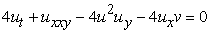(1)(2)

and(3)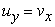(4)

and(5)(6)

eqs (1)-(6): indicate that a is scalar.

### 2. The Tan-Cot Function Method

Consider the nonlinear partial differential equation in the form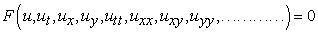(7)

where u(x, y, t) is a traveling wave solution of nonlinear partial differential equation Eq. (7). We use the transformations,(8)

Where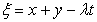(9)

This enables us to use the following changes:(10)

Using Eq. (10) to transfer the nonlinear partial differential equation Eq. (7) to nonlinear ordinary differential equation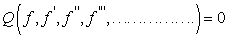(11)

The ordinary differential equation (11) is then integrated as long as all terms contain derivatives, where we neglect the integration constants. The solutions of many nonlinear equations can be expressed in the form: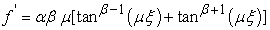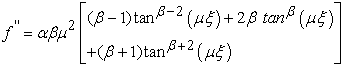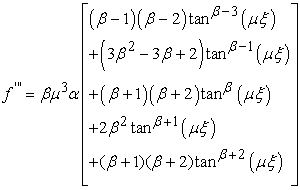(12)

and their derivatives. Or use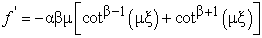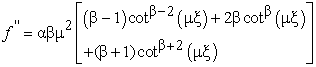(13)

and so on.

Where α, μ, and β are parameters to be determined, μ and λ are the wave number and the wave speed, respectively. We substitute Eq.(12) or Eq. (13) into the reduced equation Eq.(10), balance the terms of the tan functions when Eq. (12) are used, or balance the terms of the cot functions when Eq. (13) are used, and solve the resulting system of algebraic equations by using computerized symbolic packages. We next collect all terms with the same power in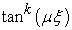or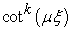and set to zero their coefficients to get a system of algebraic equations among the unknown's α, μ and β, and solve the subsequent system.

### 3. Applications

3.1. The (2+1)-Dimensional Bogoyavlenskii System

The dimensionless form of the (2+1)-dimensional Bogoyavlenskii equation that is going to be studied in this paper is given by :(14)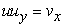Firstly, we introduce the transformation(15)

Substituting Eq. (15) into Eq. (14) and integrating once the second equation of system (14) and for simplicity, equating the integration constant equal to zero, we have(16)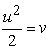The substitution of the second equation of system (16) into system (14), after integrating once the resultant, yields(17)

Substituting Eq. (12) into Eq. (17) gives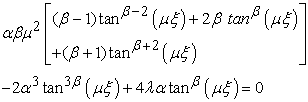(18)

Equating the exponents and the coefficients of each pair of the tan functions we find the following system of algebraic equations:(19)

Solving the system (18) yields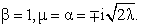(20)

Then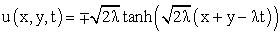(21)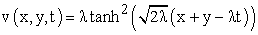(22)
3.2. Konopelchenko-Dubrovsky Coupled System(23)(24)

This system was studied by Taghizadeh et al. by applying the first integral method. Anwar used the tanh method, and sine-cosine method to solved this system.

To solve this system by using the traveling wave transformation in Eq. (9), The nonlinear system of partial differential equations Eq. (23), and Eq. (24) is carried to a system of ordinary differential equations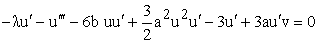(25)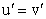(26)

Integrating Eq.(20) once with zero constant and we postulate the tanh series, Eq(20) reduces to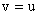(27)

Subtitute Eq.(27) in Eq.(25), then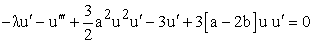(28)

Integrating Eq.(28) once with zero constant, Eq(28) reduces to(29)

Substituting Eq. (12) into Eq. (29) gives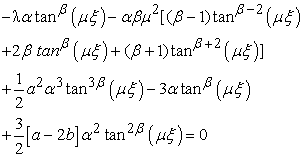(30)

Equating the exponents and the coefficients of each pair of the tan functions we find the following system of algebraic equations: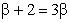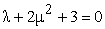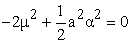(31)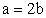Solving the system (31) yields(32)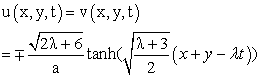(33)
3.3. The Coupled Nonlinear Evolution Equations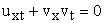(34)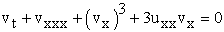(35)

This system was solved by Ahmet et al.  by applying the first integral method.

Using the transformation(36)

The system of equations (34) and (35) becomes:(37)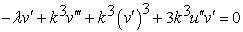(38)

From eq. (36):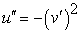(39)

Substitute (39) in (37):(40)

Assume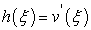(41)

then(42)

Applying Eq.(12) and assume: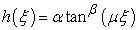(43)

Equation (42) gets the following:(44)

Equating the exponents and the coefficients of each pair of the tan functions we find the following system of algebraic equations:(45)Solving the system (45) yields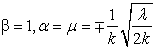(46)(47)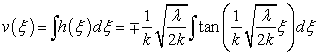(48)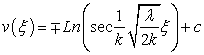(49)(50)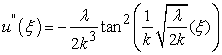(51)(52)(53)

Where: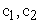are integration constants.

### 4. Results and Discussion

1. The Exact solutions for the Bogoyavlenskii equation are studied by Peng et al.  Using the traveling wave method and the singular manifold method. Results by the tan-cot method in Eq.(20), and Eq.(21) are compatible to the results calculated by Peng et al. .

2. Results in Eq.(49), and Eq.(53) are compatible with that results calculated by Ahmetet al .

### 5. Conclusion

In this paper, the Tan-Cotfunction method has been successfully implemented to establish new solitary wave solutions for various types of nonlinear PDEs. We can say that the new method can be extended to solve the problems of nonlinear partial differential equations which arising in the theory of solitons and other areas.

### References

  M Alquran, K Al-Khaled, H AnanbehStudies in Mathematical Sciences3 1 (2011).In article  W MalflietAm. J. Phys.60 650 (1992).In article  A Khater,WMalfliet, DCallebaut, and E KamelChaos Solitons Fractals14 513(2002)In article  A M Wazwaz Chaos Solitons Fractals.28454 (2006).In article  S A El-Wakil, M A AbdouChaos Solitons Fractals, 31840 (2007).In article  AJ M Jawad, S Johnson, A Yildirim, S Kumar and A Biswas Indian J Phys87287(2013).In article  A M Wazwaz Chaos Solitons and Fractals25 55(2005).In article CrossRef  T C Xia, BLi, and H Q ZhangAppl. Math. E-Notes1 139(2001).In article  E Yusufoglu, A BekirInternat. J. Comput. Math83 915 (2006).In article  M Inc, M ErgutAppl. Math. E-Notes5 89(2005).In article  Zhang,Sheng Chaos Solitons Fractals301213 (2006).In article  Z S FengJ Phys. A. Math. Gen35 343(2002).In article CrossRef  T R Ding, C Z Li Ordinary Differential Equations.Peking University Press, Peking (1996).In article  Y. Z. Peng, M. Shen Pramanajournal of physics67449(2006).In article  Taghizadeh and M. MirzazadehApplications and Applied Mathematics6 1893(2011).In article  AJ M Jawad IOSR Journal of Mathematics (IOSRJM)1 27(2012).In article  A Bekir, and ÖÜnsal Pramana journal of physics79 3(2012).In article